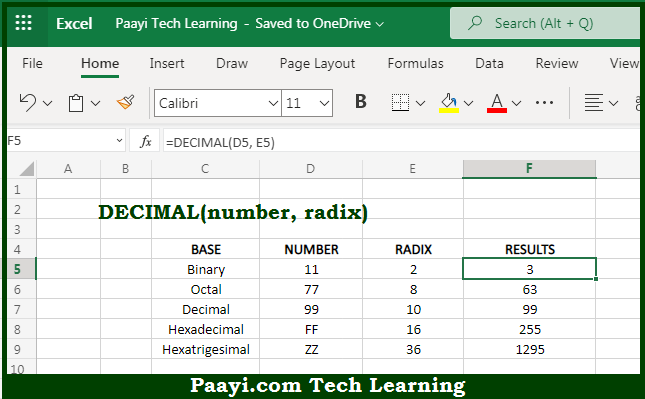# Learn How to Use Microsoft Excel DECIMAL Function

Written by | 0 Comments | 458 Views

In this article, you will learn how to use the Microsoft Excel DECIMAL function and its prime function in Microsoft Excel. You will also get to know the Microsoft Excel DECIMAL function return value and syntax with the help of some examples.

Microsoft Excel DECIMAL Function

The main function of the Microsoft Excel DECIMAL function is to convert an alpha-numeric number to a decimal. So, with the help of the DECIMAL function, you can able to return the decimal equivalent from its alpha-numeric equivalent. So, with the help of the Microsoft Excel DECIMAL function, you can easily convert the alpha-numeric number to a decimal equivalent.

Return Value of DECIMAL Function

The return value will be the decimal number.

Syntax of DECIMAL Function

Where the arguments:

• number: This is the string of alphanumeric characters.
• radix: This is the number within the range (2, 36) that represents the base of the number to be converted.

How to Use Microsoft Excel DECIMAL Function?So we know that the Microsoft Excel DECIMAL function is used to easily convert the alpha-numeric number to a decimal equivalent. It should be noted that The input string must only have the valid alpha-numeric characters corresponding with the provided base or radix. So, with the help of the Microsoft Excel DECIMAL function, you can easily return the decimal equivalent from its alpha-numeric equivalent.